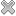# Courses / Module

Toggle Print

##MATHEMATICS I

Module code: EE106FZ
Credits: 5
Semester: 1
Department: INTERNATIONAL ENGINEERING COLLEGE
International:Overview

Indicative Syllabus • Introduction, motivation and scope of course. • Number systems • Complex numbers • Complex conjugate • Exponential and polar form • Inequalities • Sequences and series • Arithmetic and geometric series • Finite and infinite sums • Convergence • Limits and continuity • Maclaurin and Taylor series • Differential calculus • Tangents to curves • L'Hopital's rule • Sum, product, quotient and chain rules for derivatives • Critical points, maxima, minima and points of inflexion • Integral calculus • Integrals as areas and limits of sums • Definite and indefinite integrals, integration techniques • Applications of Integration • Calculation of lengths, areas and volumes • Mean and RMS values • Introduction to numerical integration • Differential equations • First order differential equations • Introduction to second order differential equationsLearning OutcomesTeaching & Learning methodsAssessmentRepeat optionsTimetable